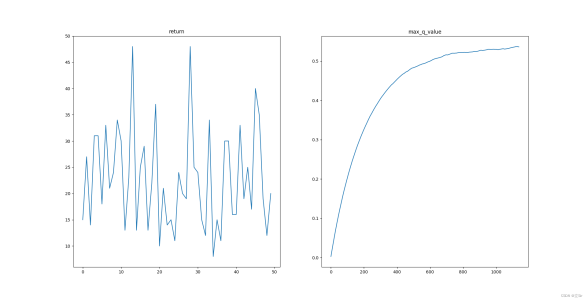https://github.com/LiSir-HIT/Reinforcement-Learning/tree/main/Model

# 1. 基于策略的深度强化学习

(1) 无法处理连续动作的任务。DQN 系列的算法可以较好地解决强化学习中大规模离散动作空间的任务，但在连续动作的任务中，难以实现利用深度神经网络对所有状态-动作的 Q 值函数近似表达。

(2) 无法处理环境中状态受到限制的问题。在基于值函数深度强化学习更新网络参数时，损失函数会依赖当前状态和下一个状态的值函数，当智能体在环境中观察的状态有限或建模受到限制时，就会导致实际环境中两个不同的状态有相同的价值函数，进而导致损失函数为零，出现梯度消失的问题。

(3) 智能体在环境中的探索性能较低。基于值函数的深度强化学习方法中，目标值都是从动作空间中选取一个最大价值的动作，导致智能体训练后的策略具有确定性，而面对一些需要随机策略进行探索的问题时，该方法就无法较好地解决。

# 2. 策略梯度法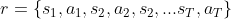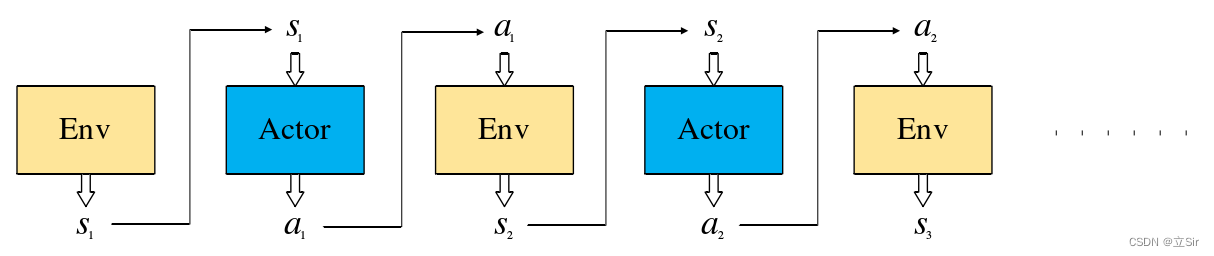# 3. 代码实现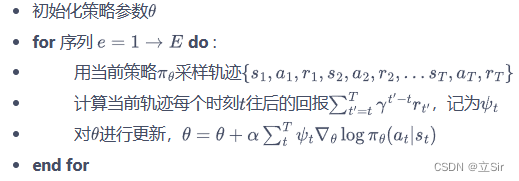1. # 基于策略的学习方法，用于数值连续的问题
2. import numpy as np
3. import torch
4. from torch import nn
5. from torch.nn import functional as F
6. # —————————————————– #
7. #（1）构建训练网络
8. # —————————————————– #
9. class Net(nn.Module):
10. def __init__(self, n_states, n_hiddens, n_actions):
11. super(Net, self).__init__()
12. # 只有一层隐含层的网络
13. self.fc1 = nn.Linear(n_states, n_hiddens)
14. self.fc2 = nn.Linear(n_hiddens, n_actions)
15. # 前向传播
16. def forward(self, x):
17. x = self.fc1(x) # [b, states]==>[b, n_hiddens]
18. x = F.relu(x)
19. x = self.fc2(x) # [b, n_hiddens]==>[b, n_actions]
20. # 对batch中的每一行样本计算softmax，q值越大，概率越大
21. x = F.softmax(x, dim=1) # [b, n_actions]==>[b, n_actions]
22. return x
23. # —————————————————– #
24. #（2）强化学习模型
25. # —————————————————– #
27. def __init__(self, n_states, n_hiddens, n_actions,
28. learning_rate, gamma):
29. # 属性分配
30. self.n_states = n_states # 状态数
31. self.n_hiddens = n_hiddens
32. self.n_actions = n_actions # 动作数
33. self.learning_rate = learning_rate # 衰减
34. self.gamma = gamma # 折扣因子
35. self._build_net() # 构建网络模型
36. # 网络构建
37. def _build_net(self):
38. # 网络实例化
39. self.policy_net = Net(self.n_states, self.n_hiddens, self.n_actions)
40. # 优化器
42. # 动作选择，根据概率分布随机采样
43. def take_action(self, state): # 传入某个人的状态
44. # numpy[n_states]–>[1,n_states]–>tensor
45. state = torch.Tensor(state[np.newaxis, :])
46. # 获取每个人的各动作对应的概率[1,n_states]–>[1,n_actions]
47. probs = self.policy_net(state)
48. # 创建以probs为标准类型的数据分布
49. action_dist = torch.distributions.Categorical(probs)
50. # 以该概率分布随机抽样 [1,n_actions]–> 每个状态取一组动作
51. action = action_dist.sample()
52. # 将tensor数据变成一个数 int
53. action = action.item()
54. return action
55. # 获取每个状态最大的state_value
56. def max_q_value(self, state):
57. # 维度变换[n_states]–>[1,n_states]
58. state = torch.tensor(state, dtype=torch.float).view(1,-1)
59. # 获取状态对应的每个动作的reward的最大值 [1,n_states]–>[1,n_actions]–>–>float
60. max_q = self.policy_net(state).max().item()
61. return max_q
62. # 训练模型
63. def learn(self, transitions_dict): # 输入batch组状态[b,n_states]
64. # 取出该回合中所有的链信息
65. state_list = transitions_dict[‘states’]
66. action_list = transitions_dict[‘actions’]
67. reward_list = transitions_dict[‘rewards’]
68. G = 0 # 记录该条链的return
70. # 梯度上升最大化目标函数
71. for i in reversed(range(len(reward_list))):
72. # 获取每一步的reward, float
73. reward = reward_list[i]
74. # 获取每一步的状态 [n_states]–>[1,n_states]
75. state = torch.tensor(state_list[i], dtype=torch.float).view(1,-1)
76. # 获取每一步的动作 –>[1,1]
77. action = torch.tensor(action_list[i]).view(1,-1)
78. # 当前状态下的各个动作价值函数 [1,2]
79. q_value = self.policy_net(state)
80. # 获取已action对应的概率 [1,1]
81. log_prob = torch.log(q_value.gather(1, action))
82. # 计算当前状态的state_value = 及时奖励 + 下一时刻的state_value
83. G = reward + self.gamma * G
84. # 计算每一步的损失函数
85. loss = -log_prob * G
86. # 反向传播
87. loss.backward()
88. # 梯度下降
89. self.optimizer.step()# 4. 实例演示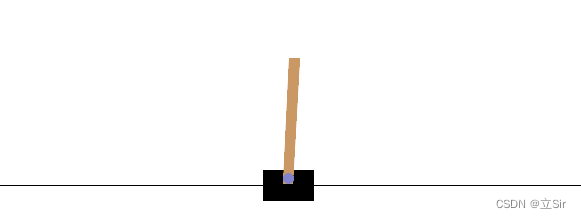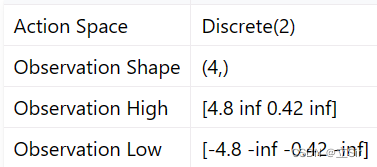1. import gym
2. import numpy as np
3. import matplotlib.pyplot as plt
5. # ——————————- #
6. # 模型参数设置
7. # ——————————- #
8. n_hiddens = 16 # 隐含层个数
9. learning_rate = 2e-3 # 学习率
10. gamma = 0.9 # 折扣因子
11. return_list = [] # 保存每回合的reward
12. max_q_value = 0 # 初始的动作价值函数
13. max_q_value_list = [] # 保存每一step的动作价值函数
14. # ——————————- #
15. #（1）加载环境
16. # ——————————- #
17. # 连续性动作
18. env = gym.make(“CartPole-v1″, render_mode=”human”)
19. n_states = env.observation_space.shape # 状态数 4
20. n_actions = env.action_space.n # 动作数 2
21. # ——————————- #
22. #（2）模型实例化
23. # ——————————- #
24. agent = PolicyGradient(n_states=n_states, # 4
25. n_hiddens=n_hiddens, # 16
26. n_actions=n_actions, # 2
27. learning_rate=learning_rate, # 学习率
28. gamma=gamma) # 折扣因子
29. # ——————————- #
30. #（3）训练
31. # ——————————- #
32. for i in range(100): # 训练10回合
33. # 记录每个回合的return
34. episode_return = 0
35. # 存放状态
36. transition_dict = {
37. ‘states’: [],
38. ‘actions’: [],
39. ‘next_states’: [],
40. ‘rewards’: [],
41. ‘dones’: [],
42. }
43. # 获取初始状态
44. state = env.reset()
45. # 结束的标记
46. done = False
47. # 开始迭代
48. while not done:
49. # 动作选择
50. action = agent.take_action(state) # 对某一状态采取动作
51. # 动作价值函数，曲线平滑
52. max_q_value = agent.max_q_value(state) * 0.005 + max_q_value * 0.995
53. # 保存每一step的动作价值函数
54. max_q_value_list.append(max_q_value)
55. # 环境更新
56. next_state, reward, done, _, _ = env.step(action)
57. # 保存每个回合的所有信息
58. transition_dict[‘states’].append(state)
59. transition_dict[‘actions’].append(action)
60. transition_dict[‘next_states’].append(next_state)
61. transition_dict[‘rewards’].append(reward)
62. transition_dict[‘dones’].append(done)
63. # 状态更新
64. state = next_state
65. # 记录每个回合的return
66. episode_return += reward
67. # 保存每个回合的return
68. return_list.append(episode_return)
69. # 一整个回合走完了再训练模型
70. agent.learn(transition_dict)
71. # 打印回合信息
72. print(f’iter:{i}, return:{np.mean(return_list[-10:])}’)
73. # 关闭动画
74. env.close()
75. # ————————————– #
76. # 绘图
77. # ————————————– #
78. plt.subplot(121)
79. plt.plot(return_list)
80. plt.title(‘return’)
81. plt.subplot(122)
82. plt.plot(max_q_value_list)
83. plt.title(‘max_q_value’)
84. plt.show()